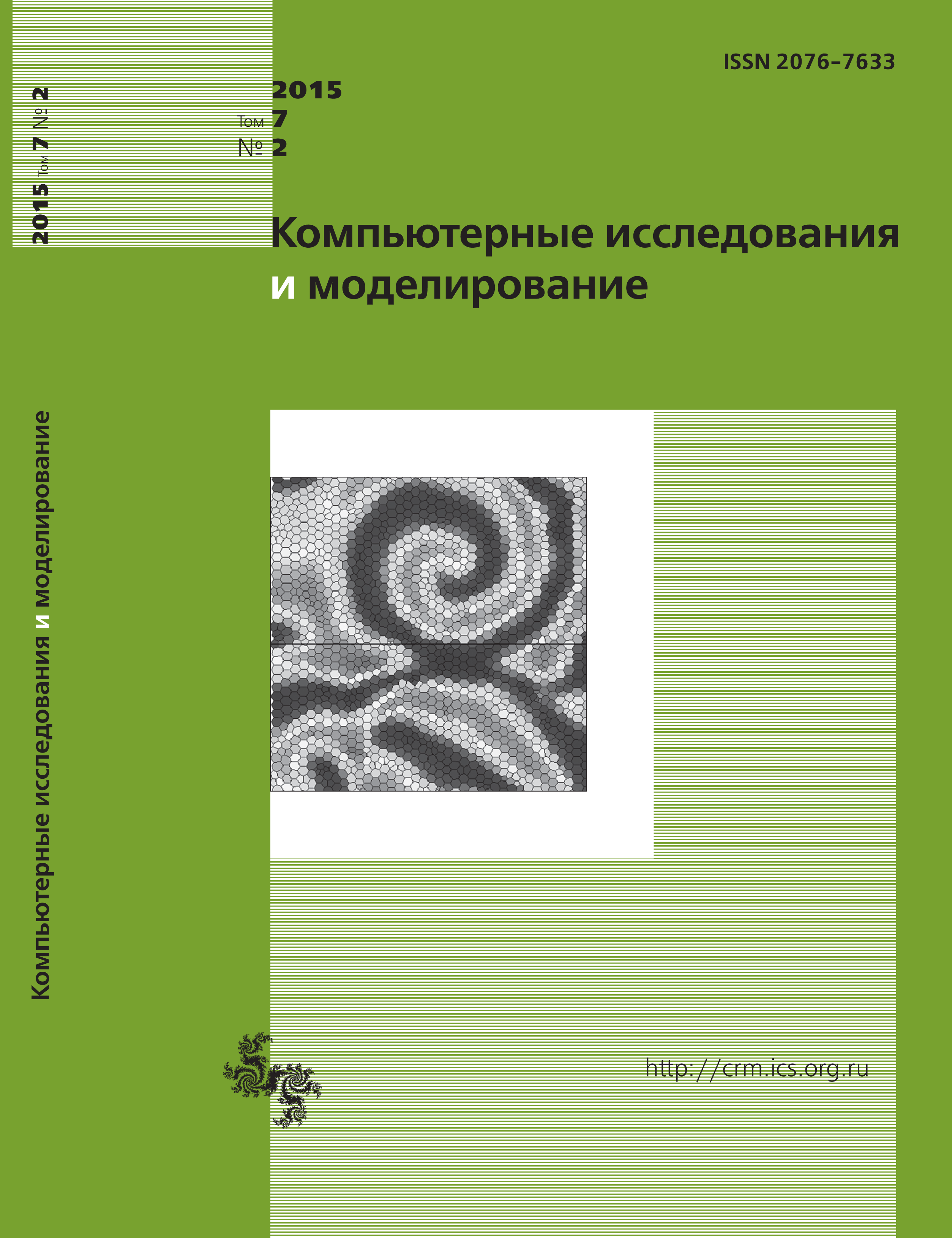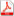Issue 2, 2015 Vol. 7

# All issues

Representation of an invariant measure of irreducible discrete-time Markov chain with a finite state space by a set of opposite directed treespdf (90K)  / List of references

A problem of finding of an invariant measure of irreducible discrete-time Markov chain with a finite state space is considered. There is a unique invariant measure for such Markov chain that can be multiplied by an arbitrary constant. A representation of a Markov chain by a directed graph is considered. Each state is represented by a vertex, and each conditional transition probability is represented by a directed edge. It is proved that an invariant measure for each state is a sum of $n^{n−2}$ non-negative summands, where $n$ is a cardinality of state space. Each summand is a product of $n − 1$ conditional transition probabilities and is represented by an opposite directed tree that includes all vertices. The root represents the considered state. The edges are directed to the root. This result leads to methods of analyses and calculation of an invariant measure that is based on a graph theory.

Keywords: Markov chain, invariant measure, directed tree
Citation in English: Krugly A.L. Representation of an invariant measure of irreducible discrete-time Markov chain with a finite state space by a set of opposite directed trees // Computer Research and Modeling, 2015, vol. 7, no. 2, pp. 221-226
Citation in English: Krugly A.L. Representation of an invariant measure of irreducible discrete-time Markov chain with a finite state space by a set of opposite directed trees // Computer Research and Modeling, 2015, vol. 7, no. 2, pp. 221-226
DOI: 10.20537/2076-7633-2015-7-2-221-226
Views (last year): 1.

Full-text version of the journal is also available on the web site of the scientific electronic library eLIBRARY.RU

The journal is included in the Russian Science Citation Index

The journal is included in the List of Russian peer-reviewed journals publishing the main research results of PhD and doctoral dissertations.

International Interdisciplinary Conference "Mathematics. Computing. Education"

The journal is included in the RSCI

Indexed in Scopus